Algebra 2 2-3 Complete Lesson: Linear Functions and Slope-Intercept Form
starstarstarstarstarstarstarstarstarstar
5 (1 rating)
by Matthew Richardson
| 25 Questions
Note from the author:
A complete formative lesson with embedded slideshow, mini lecture screencasts, checks for understanding, practice items, mixed review, and reflection. I create these assignments to supplement each lesson of Pearson's Common Core Edition Algebra 1, Algebra 2, and Geometry courses. See also mathquest.net and twitter.com/mathquestEDU.The outlined content above was added from outside of Formative.1
1
5 pts
Solve It! By what value do you multiply height h to get height H?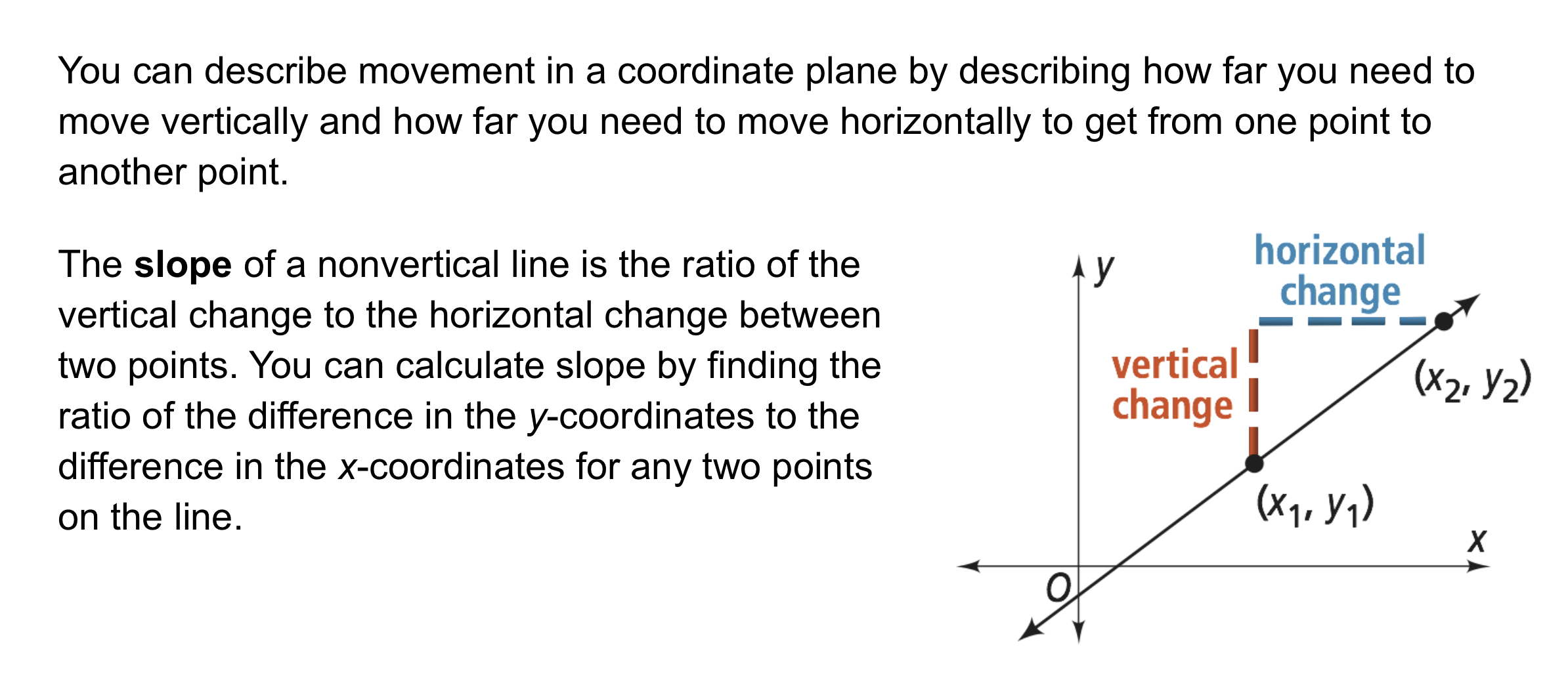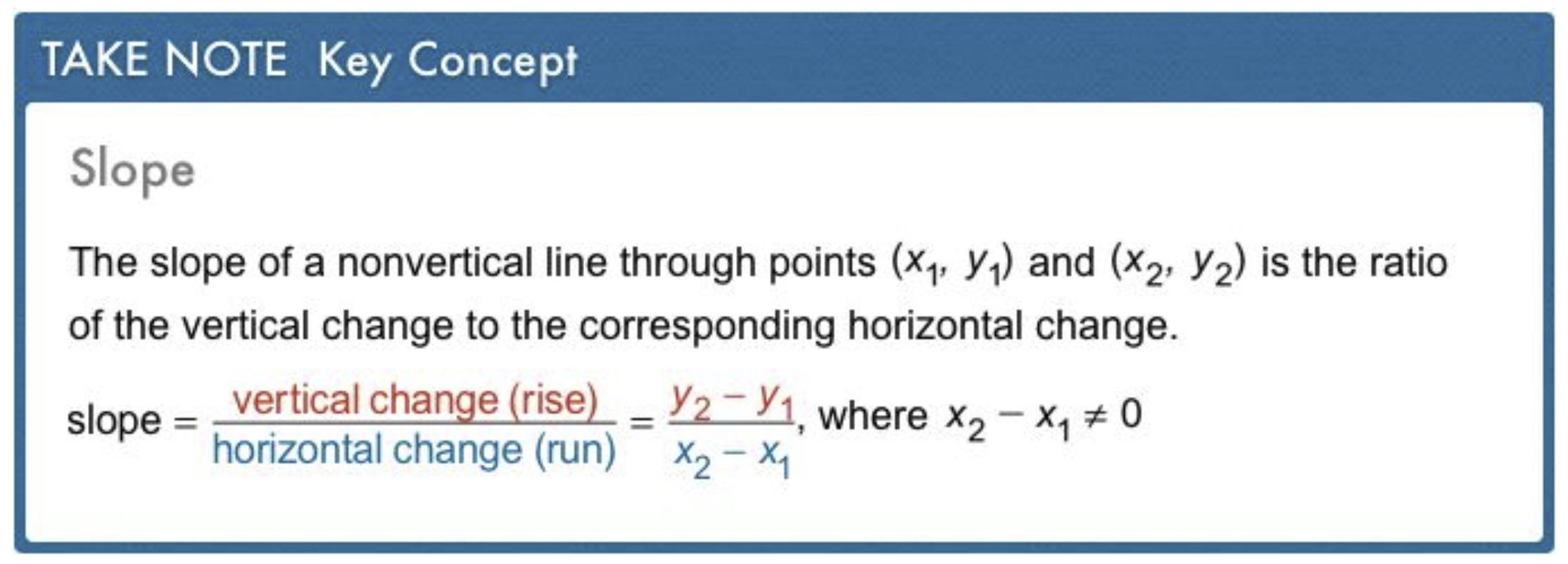2
5 pts
Problem 1 Got It? What is the slope of the line that passes through the points?
Enter only a number.
2
3
-1
1
3
5 pts
Problem 1 Got It? What is the slope of the line that passes through the points?
Enter only a number or write "Undefined"
4
5 pts
Problem 1 Got It? What is the slope of the line that passes through the points?
Enter only a number or write "Undefined"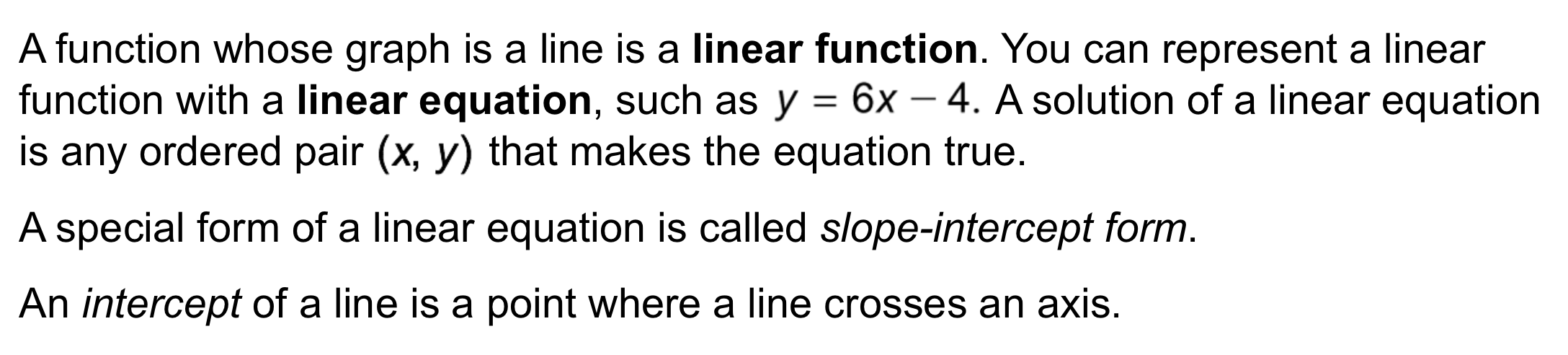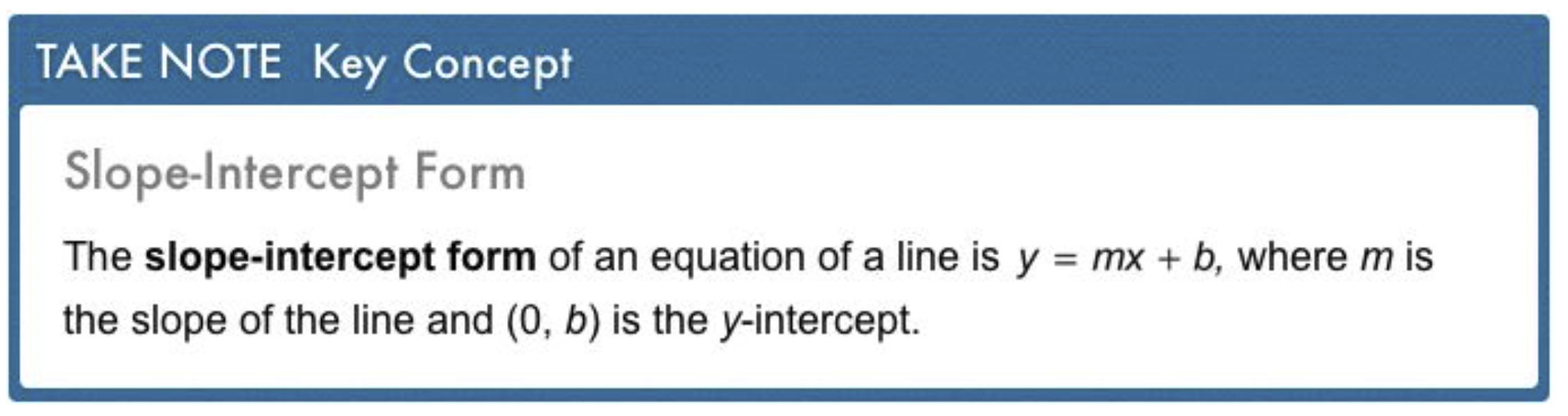5
10 pts
Problem 2 Got It? What is the equation of a line with m = 6 and y-intercept (0, 5)?
y = 6x + 5
y = 6x
y = 5x + 6
y = 5
6
10 pts
Problem 2 Got It? What is the equation of a line graphed below?

7
10 pts
Problem 2 Got It? Reasoning: In the previous item, do you get a different equation if you use (-6, 0) and the y-intercept to find the slope of the line? Explain.
Yes; choosing different points results in a different slope.
No; any two points on a line can be used to calculate the slope.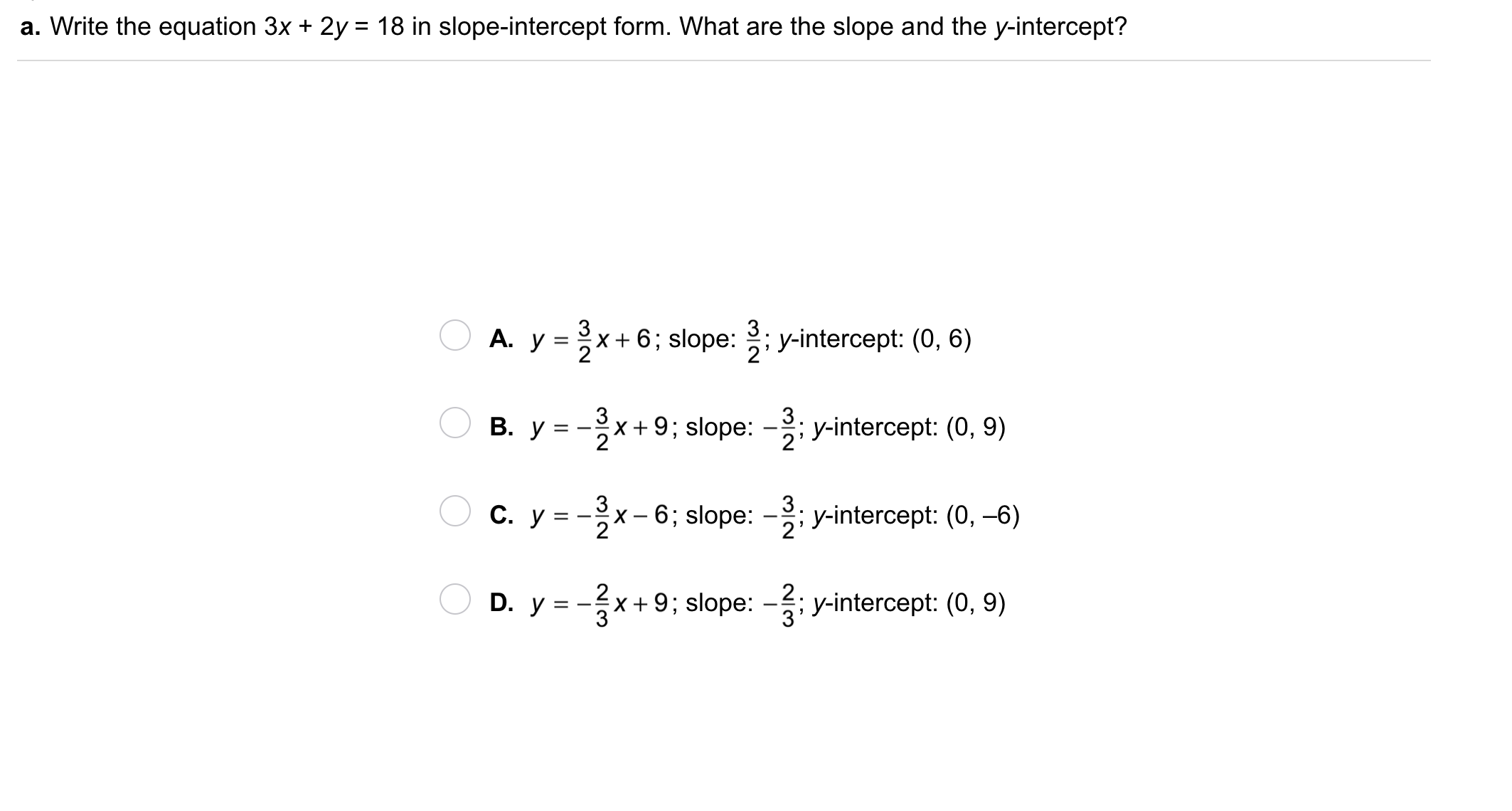8
8
10 pts
Problem 3 Got It?
A
B
C
D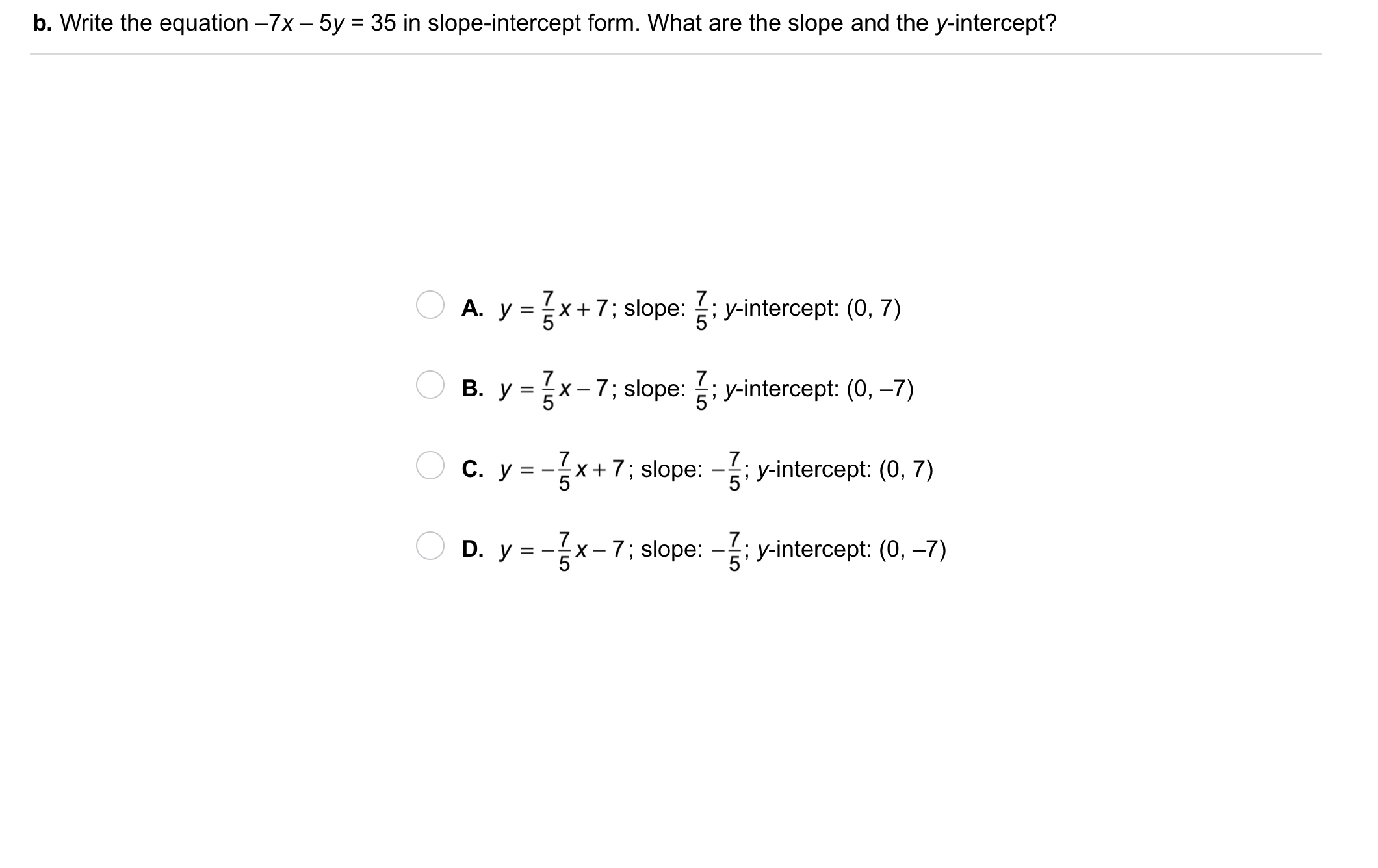9
9
10 pts
Problem 3 Got It?
A
B
C
D
10
10 pts
Problem 4 Got It? What is the graph of the equation? Hint: convert the equation to slope-intercept form.

Verification

Graph the equation from the previous item on the embedded Desmos graphing utility below. Note any differences between the Desmos graph and your graph above. Edit your graph above if necessary.
The outlined content above was added from outside of Formative.11
11
10 pts
A
B
C
D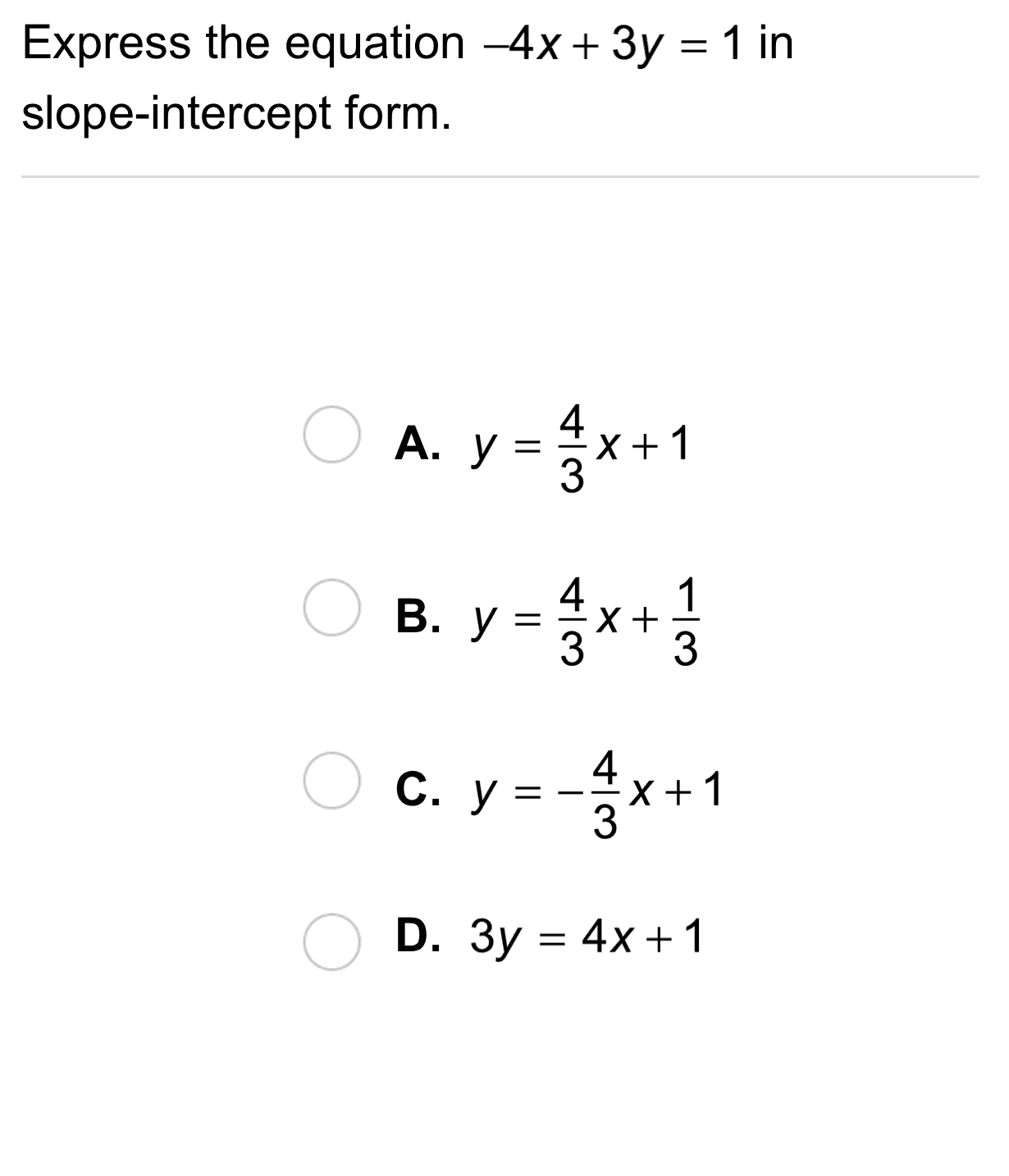12
12
10 pts
A
B
C
D13
13
10 pts
A
B
C
D14
14
10 pts
A
B
C
D15
10 pts
Vocabulary: What is a y-intercept? How is a y-intercept different from an x-intercept?
17
10 pts
Error Analysis: A classmate found the slope between two points. What error did she make?18
10 pts
Review Lesson 2-1: Match each relation with its domain.

• Domain: {-2, -1, 2, 3}
• Domain: all real numbers
• Domain: {-2, 1, 2, 3, 4}
• Domain: {-5, 0, 2, 9}
19
10 pts
Review Lesson 2-1: Match each relation with its range.

• Range: {-2, -1, 2, 3}
• Range: all real numbers y ≥ -2
• Range: {-2, 1, 2, 3, 4}
• Range: {-3, -1, 5, 15}
20
10 pts
Review Lesson 2-1: Categorize each relation as a function or NOT a function.

• Function
• NOT a function
21
10 pts
Review Lesson 1-3: Match each function with its value for x = 0.
• 5x + 2
• (x - 4) + 12
• 13 - 6.5x
• 13
• 8
• 2
• 522
10 pts
Vocabulary Review: Classify each relation based on whether or not it is a function.
• {(0, 1), (-1, 3), (0, 4)}

• Function
• NOT a function23
10 pts
Use Your Vocabulary: Use the graph below. Match each line in the left column with its slope type on the right.

• line a
• line b
• line c
• line d
• Positive slope
• Negative slope
• Zero slope
• undefined
24
100 pts
Notes: Take a clear picture or screenshot of your Cornell notes for this lesson. Upload it to the canvas. Zoom and pan as needed.

For a refresher on the Cornell note-taking system, click here.
25
10 pts
Reflection: Math Success
Add to my formatives list

Formative uses cookies to allow us to better understand how the site is used. By continuing to use this site, you consent to the Terms of Service and Privacy Policy.